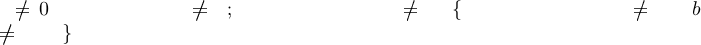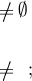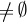Algebraic General Topology. Vol 1: Paperback / E-book || Axiomatic Theory of Formulas: Paperback / E-book

As an Amazon Associate I earn from qualifying purchases.Convergence of funcoids
by Victor Porton
October 13, 2012
Abstract
Considered convergence and limit for funcoids (a generalization of proximity spaces).
I also have dened (generalized) limit for arbitrary (not necessarily continuous) functions
under certain conditions.
Keywords: limit, convergence, discontinuous analysis, nonsmooth analysis, non smooth
analysis
A.M.S. subject classication: 54A20, 54E05, 32A70, 46F30, 49J52
1 Draft status . . . . . . . . . . . . . . . . . . . . . . . . . . . . . . . . . . . . . . . . . . . . . . . . . 1
2 Common . . . . . . . . . . . . . . . . . . . . . . . . . . . . . . . . . . . . . . . . . . . . . . . . . . . 1
3 Convergence . . . . . . . . . . . . . . . . . . . . . . . . . . . . . . . . . . . . . . . . . . . . . . . . . 1
4 Limit . . . . . . . . . . . . . . . . . . . . . . . . . . . . . . . . . . . . . . . . . . . . . . . . . . . . . . 2
5 Generalized limit . . . . . . . . . . . . . . . . . . . . . . . . . . . . . . . . . . . . . . . . . . . . . 2
5.1 The deﬁnition . . . . . . . . . . . . . . . . . . . . . . . . . . . . . . . . . . . . . . . . . . . . . . 2
5.2 Generalized limits as a generalization of limits . . . . . . . . . . . . . . . . . . . . . . . . . 4
5.3 Yet to do . . . . . . . . . . . . . . . . . . . . . . . . . . . . . . . . . . . . . . . . . . . . . . . . . 4
Bibliography . . . . . . . . . . . . . . . . . . . . . . . . . . . . . . . . . . . . . . . . . . . . . . . . . . 4
1 Draft status
This is a partial draft.
2 Common
See  for the deﬁniti on of funcoid.
3 Convergence
[TODO: Specify sources and destinations of the considered funcoids and reloids.]
Deﬁnition 1. A ﬁlter object F converges to a lter object A regarding a funcoid µ (F
µ
A) iﬀ
F hµiA.
1
Deﬁnition 2. A funcoid f conv erges to a ﬁlt er object A regarding a funcoid µ (f
µ
A) iﬀ im f
hµiA that is iﬀ im f
µ
A.
. This document has been written using the GNU T
E
X
MACS
text editor (see www.texmacs.org).
1. This generalizes the standard denition of lter convergent to a point or to a set.
1Deﬁnition 3. A funcoid f converges t o a ﬁlter object A on a ﬁlter object B regarding a funcoid µ
iﬀ f |
B
µ
A.
Remark 4. We can deﬁne also convergence for a reloid f : f
µ
A im f hµiA or what is the
same f
µ
A (FCD)f
µ
A.
Theorem 5. Let f, g, µ, ν are funcoids, A is a ﬁlter object. If f
µ
A,
g|
hµiA
C(µ (hµiA)
2
; ν)
and hµiA A then g f
ν
hgiA.
Proof. im f hµiA; hgiim f hgihµiA; im(g f) hg|
hµiA
ihµiA; im(g f) hg|
hµiA
ihµ
(hµiA)
2
iA; im(g f) hg|
hµiA
(µ (hµiA)
2
)iA; im(g f) hν g|
hµiA
iA; im(g f) hν giA;
im(g f) hν ihgiA; g f
ν
hgiA.
Corollary 6. Let f, g, µ, ν are funcoids, A is a ﬁlter object. If f
µ
A, g C(µ; ν) and hµiA A
then g f
ν
hgiA.
Proof. Fro m th e last theo rem and a theorem in .
The following is the theorem about convergence of a continuous funcoid:
Theorem 7. If f C(µ; ν) then f |
hµiA
ν
hf iA (for any funcoids µ and ν and a ﬁlter object A).
Proof. f |
hµiA
ν
hf iA im f |
hµiA
hν ihf iA hf ihµiA hν ihf iA hf µiA hν f iA
f µ ν f f C(µ; ν).
4 Limit
Deﬁnition 8. lim
µ
f = a iﬀ f
µ
{a} for a T
2
-separable funcoid µ and a non-empty funcoid f.
It is deﬁned correctly, that is f has no more than one limit.
Proof. Let lim
µ
f = a and lim
µ
f = b. Then im f hµi{a} and im f hµi{b}.
Because f
0 we have im f
0; hµi{a} hµi{b}
0; {b} hµ
1
ihµi{a}
0; {b}
hµ
1
µi{a}
0; {a} [µ
1
µ] {b}. Because µ is T
2
-separable we have a = b.
Deﬁnition 9. lim
B
µ
f = lim
µ
(f |
B
).
Remark 10. We can also in an obvious way deﬁne limit of a reloid.
5 Generalized limit
5.1 The deﬁnition
Let µ and ν are func oids , G is a group of functions.
Let D is a set such that r G: im r D x, y Dr G: r(x) = y.
We require that µ and any r G commute, t hat is µ r = r µ.
We require for every y
ν hν i{y} ×
FCD
hν i{y} (1)
Remark 11. The formula (1) usually works if ν is a proximity. It does not work if µ is a
pretopology or preclosure.
2 Section 5We are going to consider (gene ralized) limits of arbitrary functions acting from µ to ν. (The
functions in consideration are not required to be continuous.)
Remark 12. Most typically G is the group of trans lations of some topological vector space.
Generalized limit is deﬁned by the following formula:
Deﬁnition 13. xlim f =
def
{ν f r | r G} for any funcoid f.
Remark 14. Generalized limit technically is a set of funcoids (see ).
We will assume that the function f is deﬁned on hµi{x}.
Deﬁnition 15. xlim
x
f =
def
xlim f |
hµi{x}
.
Obvious 16. xlim
x
f = {ν f |
hµi{x}
r | r G}.
Remark 17. xlim
x
f is the same for funcoids µ and Compl µ.
Lemma 18. (A ×
FCD
B) f = hf
1
iA ×
FCD
B for every f FCD, A, B F.
Proof. For every ﬁlter object X
h(A ×
FCD
B) f iX =
h(A ×
FCD
B)ihf iX =
B if hf iX A
;
if hf iX A = ;
=
(
B if X hf
1
iA
;
if X hf
1
iA = ;
=
hhf
1
iA ×
FCD
BiX .
The function τ will deﬁne an injection from the set of points of the space ν (“numbers” , “points”,
or “vectors”) to the set of all (generalized) limits (i.e. values which xlim
x
f may take).
Deﬁnition 19. τ(y) =
def
{hµi {x} ×
FCD
hν i{y } | x D}.
Proposition 20. τ(y) =
def
{(hµi{x} ×
FCD
hν i{y }) r | r G} for every x D.
Proof. (hµi{x} ×
FCD
hν iy) r = hr
1
ihµi{x} ×
FCD
hν iy = hµihr
1
i{x} ×
FCD
hν iy =
hµi{ r
1
x} ×
FCD
hν iy {hµi{x} ×
FCD
hν i{y } | x D} where x
D.
Reversely hµi{x} ×
FCD
hν i{y } = (hµi{x} ×
FCD
hν i{y }) e where e is the identify element of
G.
Theorem 21. If f |
hµi{x}
C(µ; ν) and hµi{x} { x} then xlim
x
f = τ (fx ).
Proof. f |
hµi{x}
µ ν f |
hµi{x}
ν f ; thus hf ihµi{x} hν ihf i{x}; consequ ently we have
ν hνihf i{x} ×
FCD
hν ihf i{x} hf ihµi{x} ×
FCD
hν ihf i{x}.
ν f |
hµi{x}
(hf ihµi{x} ×
FCD
hν ihf i{x}) f |
hµi{x}
=
h(f |
hµi{x}
)
1
ihf ihµi{x} ×
FCD
hν ihf i{x}
dom f |
hµi{x}
×
FCD
hν ihf i{x} =
hµi{ x} ×
FCD
hν ihf i{x}.
Generalized limit 3im(ν f |
hµi{x}
) = hν ihf ihµi{x};
ν f |
hµi{x}
hµi{x} ×
FCD
im(ν f |
hµi{x}
) =
hµi{x} ×
FCD
hν ihf ihµi{x}
hµi{ x} ×
FCD
hν ihf i{x}.
So ν f |
hµi{x}
=hµi{x} ×
FCD
hν ihf i{x}.
Thus xlim
x
f = {(hµi{x} ×
FCD
hν ihf i{x}) r | r G} = τ(fx).
Remark 22. Without the requirement of hµi{x} {x} the last theorem would not work in the
case of removable singularity.
Theorem 23. Let ν ν ν. If f |
hµi{x}
ν
{y} then xlim
x
f = τ (y).
Proof. im f |
hµi{x}
⊆hν i{y }; hf ihµi{x} hν i{y};
ν f |
hµi{x}
(hν i{y} ×
FCD
hν i{y }) f |
hµi{x}
=
h(f |
hµi{x}
)
1
ihν i{y} ×
FCD
hν i{y } =
hI
hµi{x}
f
1
ihν i{y} ×
FCD
hν i{y }
hI
hµi{x}
f
1
ihf ihµi{x} ×
FCD
hν i{y } =
hI
hµi{x}
ihf
1
f ihµi{x} ×
FCD
hν i{y }
hI
hµi{x}
ihI
hµi{x}
ihµi{x} ×
FCD
hν i{y } =
hµi{x} ×
FCD
hν i{y}.
On the other hand, f |
hµi{x}
⊆hµi{x} ×
FCD
hν i{y};
ν f |
hµi{x}
⊆hµi {x} ×
FCD
hν ihν i{y} hµi{x} ×
FCD
hν i{y }.
So ν f |
hµi{x}
=hµi{x} ×
FCD
hν i{y}.
xlim
x
f = {ν f |
hµi{x}
r | r G} {(hµi{x} ×
FCD
hν i{y }) r | r G} = τ(y).
Corollary 24. If lim
hµi{x}
ν
f = y then xlim
x
f = τ (y).
We have bijective τ if hν i{y
1
}
F
hν i{y
2
}
that is if ν is T
1
-separable.
5.2 Generalized limits as a g eneralization of limits
When τ is bijective, using the procedure des cribed in appendix B in  we can equ ate points of
the space with certain generalized limits.
Thus we can use only “lim to denote all kinds of limits and eliminate “xlim” notation.
5.3 Yet to do
We need to study generalized limits of composition of functions. It is yet unclear how to deﬁne this.
We should introduce n-ary functions extended on values which generalized limits take, so that
we could be able for example to add two generalized limits.
We should study diﬀerential equations genera lized for the derivative of non-s mooth functions.
Need examples with metric spaces.
Bib liography
 Victor Porton. Filters on posets and generalizations. At http://www.mathematics21.org/binaries/l-
ters.pdf.
 Victor Porton. Funcoids and reloids. At http://www.mathematics21.org/binaries/funcoids-reloids.pdf.
4 Section
 Victor Porton. Generalized continuousness. At
http://www.mathematics21.org/binaries/continuousness.pdf.
Bibliography 5